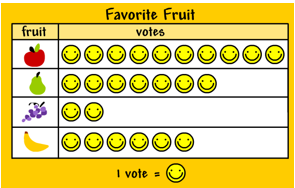Mathematics
Easy

Question

# The number of votes for pears is ______.## 5247Hint:

## The correct answer is: 7

###Here we have to find the number of votes for pears,Firstly, In fig, we are given by two column . One is fruit and another is votes,Look pears in fruit column , It is on 2nd place so,Count the total number of votes in same row of pears, Therefore, the total number votes for pears is 7.The correct answer is 7 (option (d)).

In this question , We have given by table we just find out the votes of the given fruit  so count the number of votes which is given in the row.

### Related Questions to study#### With Turito Foundation.#### Get an Expert Advice From Turito.